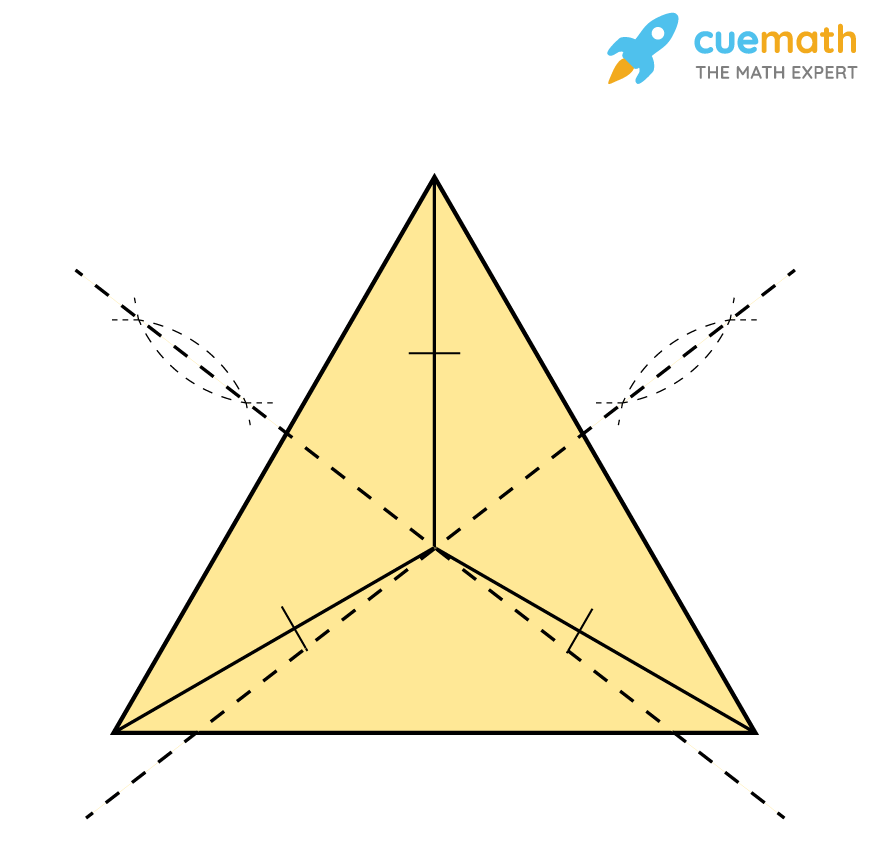# What Is the Circumcenter of a Triangle

## Question: What is the circumcenter of a triangle?

polygon with three vertices and three edges is called a triangle.

## Answer: The circumcenter is the intersection point of all three perpendicular bisectors of a triangle.

Let us explore more about the circumcentre of a triangle.

## Explanation:

The circumcenter is the center point of the circumcircle drawn around a polygon.

The circumcenter of a triangle can be found out as the intersection of the perpendicular bisectors.• The circumcenter of a triangle is denoted by S.
• By the position of the circumcenter, we can understand the nature of the given triangle.
• A circumcenter is equidistant from all the three vertices of the triangle.